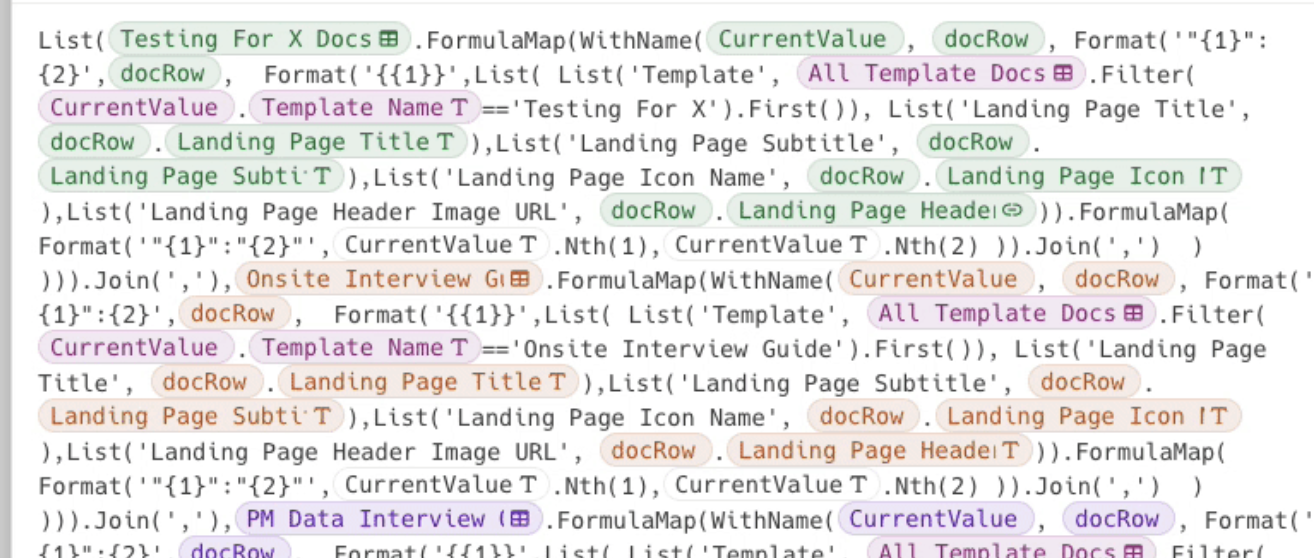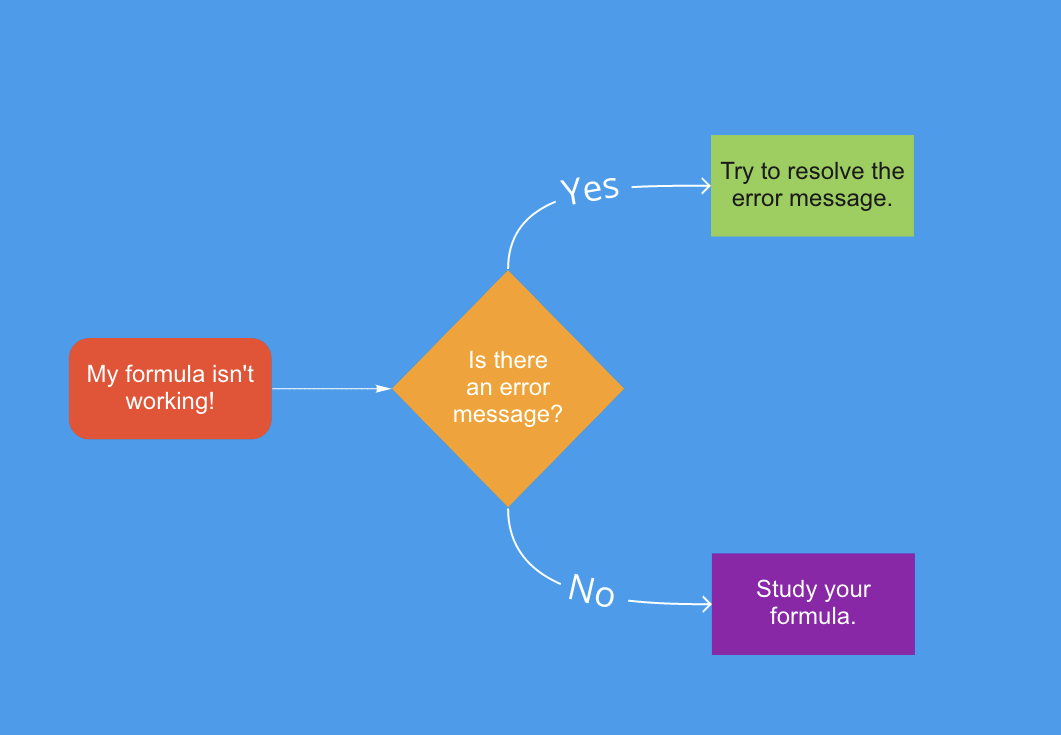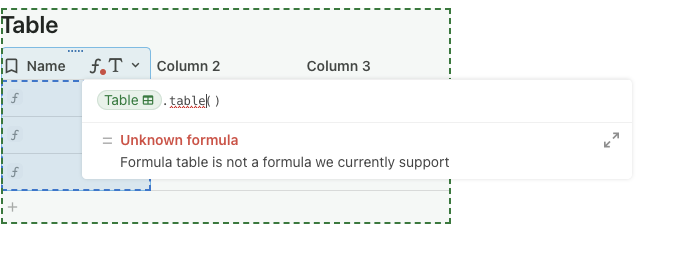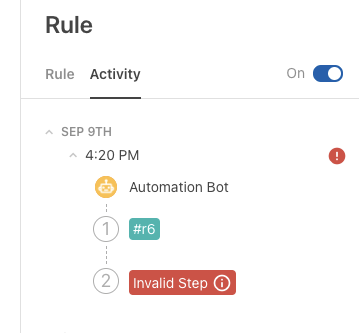One Codan's guide on how to debug formulas
Share
Explore# Introduction

Formulas are a powerful part of the Coda experience. They allow Coda to perform more complex actions ranging from logic based filtering of data to automating away tedious actions. But as most makers find out, writing formulas can sometimes be tricky. This article is going to try and help with that.

Note: this is my personal framework for working with Coda
; I use these two questions as guides for how to tackle the work I need to do on a day to day basis but you may prefer a different approach 👍

But before we get into tips about how to debug a Coda formula, we want to point out a few things this doc
won’t
cover:

To state the affirmative: this article is for folks who know how to write a Coda formula but wish for some general guidance around debugging a formula. Let’s get to it! 😃

To summarize how I think about debugging a Coda formula, I’m going to use this diagram:Let’s walk through the different parts of the diagram.

# My Formula Isn’t Working!

This is probably the part you don’t need help with but let’s still talk through it! 😅

A red squiggly line. A red rectangle showing “Error”. The formula doing the right thing in the wrong place. The formula doing nothing at all.

These and many other scenarios are all ways to make us say “ugh this formula isn’t working”. But what’s important is that we pay attention to
how
the formula is misbehaving. I stress this because paying attention to this helps us solve the formula error.

Coda formulas can fail for a variety of reasons, which means there are also a variety of solutions. To help us figure out which solution is appropriate, we need to pay attention to what is going on with the particular formula we’re looking at.

To make things a little bit easier though, I’ve tried to some up with a single question and two “paths” based on the answer to that question.

# The Question: Is there an error message?

Why is this
the
question? Because if there’s a Coda error message, it usually contains specific information that will help us fix our formula faster. Coda error messages usually look like this:Basically, if you’re seeing
red
in or around your formula, something is probably off. But not to worry, the error indicators usually include a helpful bit of information. In the first image, there’s some text beneath the formula indicating what is wrong. In the second image, which is a picture of an automation, if you mouse over the actual “Invalid Step” box (but this is just an image, so imagine you’re doing it for real!) you would see a little pop-up message explaining the error.

Curious about all the different Coda formula errors? Here’s a list!

### Error/Warning Messages in formulas todayError/Warning Messages in formulas today0

But let’s walk through some live examples.

# “Yes, there’s an error message.”

## Example 1: Wrong Argument Type

Formula:
[ ]

Let’s (right) click into the formula to open the formula editor. There should be an error message.

It says
Sum()
expects to act on a number or list of numbers. If we look at the rest of the formula, it becomes understandable why the formula returns this error.

Examine this formula:
@Dex’s Diner
@O.K. Corral
@The Winchester Tavern
. All I did was remove the
Sum()
from the end of the earlier formula.

If we look at the output, it’s outputting a list of
row objects
, not a number!

We can correct this by using dot notation to project out the
Revenue
column:
\$134,651.00

## Example 2: Circular Reference

Take the table below. Click on the cursive “F” with a “T” icon/button in the
Cost
column and inspect the formula (following the red dot!).

You should see a red underline with an error message suggesting that this formula is a
.
4
Cost
1
2
3
4
There are no rows in this table

To “fix” this, we should delete the
Average()
formula. Cool, we’re done here. I’ll see ya tomorrow...

Haha just kidding! You might be wondering “hey wait, Zac how am I supposed to calculate the average of the
Cost
column?”

That’s where this question becomes fun. Unlike the last formula, we can’t correct it by editing the formula in the same place. To make things more fun: there’s more than one way to get the average of the
Cost
column. To keep things simple, I’ll say there are three ways:
Use the “Summarize” feature in the column header and summarize by average.
Write a formula like
in the canvas.
Write a formula like
in a different table.

But which of these should you use? And why does it feel like this question got more complicated?

To answer the second question: because now we’re introducing a two-part question:
What is it that I’m trying to do?
If I know what I’m trying to do, how do I make it happen in Coda?

Stepping back for a second,
this is my personal framework for working with Coda
; I use these two questions as guides for how to tackle the work I need to do on a day to day basis!

And this is the framework I would recommend you use to decide which approach is best for you, as each of those three answers has different implications. To keep running with this example:
Use the “Summarize” feature in the column header and summarize by average
Gets you the number without writing a formula but you won’t be able to access this number anywhere else in the doc or have it appear if you email the table out.
Write a formula like
in the canvas.
Returns the number, but if you wanted to use it against data in another table you probably want to go with the next option. An example would be comparing how much an individual business’ cost compares to the average cost.
Write a formula like
in a different table.
More work if you don’t need it in a table. Also, you’ll notice it returns the same number for all cells... does that really make sense?

Three different choices, with different implications. If I knew what I was trying to do, I’d know which approach to take.

But what if you’re not seeing an error message?

# “No, there isn’t an error message!”

To state the obvious: things get a bit trickier when there isn’t an error message because we don’t have a simple pointer on what’s wrong with a formula.

To say something less obvious, there isn’t an error message because things are “working”, just not working as you intended. But we’re not completely lost! Let’s go through some tips about how to “fix” formulas without error messages.

I actually like to start by double checking if my formula is working as intended. What I mean is that, the formula is actually doing what I want it to do but I haven’t yet recognized that myself. If that was confusing, let me give an example.

## Example 1: No rows returned.

Take the below table. It starts off having no rows.
Receiving Table
0
Account
Revenue
Cost
There are no rows in this table

Now let’s take this button.
Click me
Try pushing it.

You’ll see it creates a row in the above receiving table but they’re all blank. Right click into the button to see the formula. Can you tell what’s wrong? In case you cannot, I’ve copied the formula below and highlighted the part that is causing the blanks.

`AddRow(``Receiving Table,``Receiving Table.Account,``Business Data.Filter(Revenue > 100000).Account,``Receiving Table.Revenue,``Business Data.Filter(Revenue > 100000).Revenue,``Receiving Table.Cost,``Business Data.Filter(Revenue > 100000).Cost``)`

Revenue > 100000
is causing there to be blanks because when this formula runs, it tries to filter the
table to a row where
Revenue
is greater than \$100,000. Well, there aren’t any rows where the value of
Revenue
is greater than \$100,000. So, the formula is actually working as intended! We just didn’t realize it.

And this leads me to reiterating my earlier point: if there isn’t an error message, it’s possible that the formula is working fine but was not written to do what we intended. In this case, I don’t think the button author intended to use a logic that would filter out all the rows and return nothing.

## Example 2: It’s formulas all the way down

For the sake of learning, let’s write a formula that’s a little bit complicated.

We want the formula to do the following:
for the rows in
Revenue Categories
where the
Revenue
is more than \$1
go through each row and check if
Revenue
is greater than \$46,000
if it is greater than \$46,000, modify the value of the
Revenue
column cell to be “High Revenue”
Revenue Categories
4
Account
Revenue
Revenue Designation
1
Mom and Pop shop
\$50,000.00
2
Dex’s Diner
\$56,223.00
3
O.K. Corral
\$45,098.00
4
The Winchester Tavern
\$33,330.00
There are no rows in this table

Following that logic would lead us to expect the table to look like the following after we’ve run the formula:
1
Account
Revenue
Revenue Designation
1
Mom and Pop shop
\$50,000.00
High Revenue
2
Dex’s Diner
\$56,223.00
High Revenue
3
O.K. Corral
\$45,098.00
Low Revenue
4
The Winchester Tavern
\$33,330.00
Low Revenue
There are no rows in this table

But what about the formula itself? What does that look like? For the sake of this exercise we’ll assume you did the prerequisite learning and understand the basics of Coda formulas.

That’s a long way of saying, here’s the formula inside this button:
right click me and then click into 'Action' to see the formula

Try clicking on it and seeing if it leads to the correct answers (you will need to reference the
Revenue Categories
view above).

Now before you go ahead and reach out to the support team or post in the community:
the button should not do anything when you click it
. Those of you who read the formula closely may know why. But the point of this exercise is to learn and apply formula debugging tips. So let’s do that!

### Tip #1: Break apart your formula into chunks.

This formula is comprised of multiple formulas. To make this a bit easier to grasp, I’ve highlighted each formula with a different color:

`Business Data.Filter(Revenue < 1).FormulaMap(`` ModifyRows(CurrentValue,Revenue Designation, `` If(`` CurrentValue.Revenue > 46000, `` "Low Revenue", `` "High Revenue"``)))`

A structured way to solve for malfunctioning formulas is to break them apart into smaller pieces and confirm that each individual part is functioning as intended.

A lot easier to read than the whole formula right? That’s the point! Breaking apart your formula into smaller pieces makes it easier to focus and troubleshoot by limiting how much you have to focus on at once.

Now let’s see what this first part actually produces on its own:
[  ]

It’s blank! But if we look at just that first part, it becomes obvious what the issue is: we’re checking if Revenue
is less than
\$1! And looking at the data, it starts to make sense why nothing is being returned; all the values in the
Revenue
column are clearly much larger than \$1. Also, we want to check if the
Revenue
is greater than \$1! So let’s change that:
@The Winchester Tavern
@O.K. Corral
@Mom and Pop shop
@Dex’s Diner

Great! Now we actually have some rows. Let’s try modifying the button formula pressing it again (I’ve re-pasted it here):
right click me and then click into 'Action' to see the formula

Revenue Categories 2
4
Account
Revenue
Revenue Designation
1
Mom and Pop shop
\$50,000.00
2
Dex’s Diner
\$56,223.00
3
O.K. Corral
\$45,098.00
4
The Winchester Tavern
\$33,330.00
There are no rows in this table

Cool values were actually filled in this time. But if we look closely, we’ll see that the
Revenue Designation
values are not quite correct. The values
below
\$46,000 are incorrectly labeled as
High Revenue
while the values
above
\$46,000 are labeled as
Low Revenue
.

Continuing the trend of breaking apart our formula into chunks. Let’s look at the whole thing again:

`Business Data.Filter(Revenue < 1).FormulaMap(`` ModifyRows(CurrentValue,Revenue Designation, `` If(`` CurrentValue.Revenue > 46000, `` "Low Revenue", `` "High Revenue"``)))`

So we know the blue part works now. And the
FormulaMap()
part seems to work. But let’s look at that
ModifyRows()
section. ModifyRows() has three inputs:
the row(s) to modify
the column to modify in the aforementioned row
the value to insert

If we think of each of the above as parts of the ModifyRows() formula, it seems that the first two parts work. We do see that the Revenue Designation column in the rows are being modified. But the value being inserted is wrong! And it’s another formula, so let’s look at it!

`If(`` CurrentValue.Revenue > 46000, `` "Low Revenue", `` "High Revenue"``)`

Again, looking at a specific part of the formula makes it easier to read for errors. And in this case, it looks like we just mixed up which value to output if the condition was
true
or
false
. So if we swap that around like so:

`If(`` CurrentValue.Revenue > 46000, `` "High Revenue",`` "Low Revenue" ``)`

the larger formula will look like:

`Business Data.Filter(Revenue < 1).FormulaMap(`` ModifyRows(CurrentValue,Revenue Designation, `` If(`` CurrentValue.Revenue > 46000, `` "High Revenue", `` "Low Revenue"``)))`

which I’ve put into this button here:
right click me and then click into 'Action' to see the formula

Click it and see what happens to the values in the view below:
Revenue Categories 3
4
Account
Revenue
Revenue Designation
1
Mom and Pop shop
\$50,000.00
2
Dex’s Diner
\$56,223.00
3
O.K. Corral
\$45,098.00
4
The Winchester Tavern
\$33,330.00
There are no rows in this table

And comparing that to the answer key:
View of Revenue Categories - Answer Key
1
Account
Revenue
Revenue Designation
1
Mom and Pop shop
\$50,000.00
High Revenue
2
Dex’s Diner
\$56,223.00
High Revenue
3
O.K. Corral
\$45,098.00
Low Revenue
4
The Winchester Tavern
\$33,330.00
Low Revenue
There are no rows in this table

It looks like the button works as intended now! Hurray!

# Closing Remarks

The tips we covered in this article might still not be enough to help you troubleshoot every formula but it’s a solid starting point that leverages the same approaches members of the Coda team use when we troubleshoot the docs of our makers, and our own!